### Day 03 - Radical Properties - 08.20.14

 Bell RingerBulldog HourPresentationRoom C220Teacher ScriptGo over previous Exit Ticket.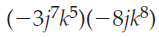-24j8k1324j7k13-11j8k1324j8k13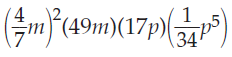4m3p68m3p65m3p68m3p5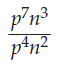p3np-3np3n0p3n2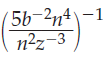b2 / (5n2z3)b / (5nz3)b2 / (5n2z)b3 / (n2z3)ReviewExponent PropertiesProduct of PowersPower of a PowerPower of a ProductQuotient of PowersPower of a QuotientZero ExponentNegative ExponentsLessonRadical PropertiesProduct of Square RootsQuotient of Square Rootspage 590 #15-23 (odds)Exit Ticket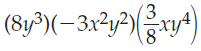-9x3y99x3y8-9x3y89x2y9none of the above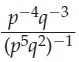p / qp2 / qp / q2p2 / q3none of the above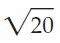2 * square root of 55 * square root of 25 * 45 * square root of 4none of the above Lesson ObjectivesHow can exponent properties be applied to solve exponent problems?Standard(s)N.RN.1  Explain how the definition of the meaning of rational exponents follows from extending the properties of integer exponents to those values, allowing for a notation for radicals in terms of rational exponents.N.RN.2  Rewrite expressions involving radicals and rational exponents using the properties of exponents.A.CED.1 Create equations and inequalities in one variable and use them to solve problems.Include equations arising from linear and quadratic functions, and exponential functions.Mathematical Practice(s)#7: Look for and make use of structureStudents will see the relationship between radical and exponential notation.